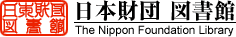#3.3 Annual serial voyage simulation (ASVS) 　 Main flow In the annual serial voyage simulation (ASVS), the total cargo amounts and freight cost for serial operation running between transit route throughout the year are calculated when the serial operation is performed in the transit routes for the period of one year. The total cargo amounts and freight costs is compared with those through the Suez route. Fig.3.3.1 represents the main flow of ASVS. 　 Serial voyage simulation The major difference between ASVS and MVS is the calculation method in the VOYAGE SIMULATION module. In MVS, operation performance for one-voyage is calculated separately in each month and in the same direction. On the other hand, in ASVS, each operation is started from the next day of the end of the previous voyage and in the opposite direction, which is repeated for one year. The beginning of the voyage is assumed the first of January, and a voyage simulation is repeated until the arrival is 31st of December or after the day. The direction of first voyage is assumed from Yokohama to Hamburg. The flow of one-voyage simulation is same as one of MSV, which is represented in Figure 3.2.3. In ASVS, the voyage is likely started in the middle of month, so the shift of month during one voyage occurs frequently. In such a case of ASVS, also the environmental data is renewed to one of the next month at the route segment where the month changes. 　 Annual cost Figure 3.3.2 illustrates the flow of ASVS cost calculation. In ASVS, the one-voyage cost is totaled separately in each cost component throughout the year. The calculation methods for cost component have been explained in chapter 3.1. As the explanation in chapter 3.2.2, the last voyage in that year would be extended over the next year. Therefore, the cost and cargo capacity are converted to one year basis, and the freight rate and a number of voyages are derived. 　 Results output The output results of the calculation in ASVS are listed below. - Stochastic value of annual cargo amount - Change of voyage-days for one year - Stochastic value of annual total cost - Stochastic value of cost component for one year - Stochastic value of freight cost - Stochastic value of cost component for one year 　 Each stochastic values above are output by the mean value combination of each voyage, the upper expected value combination of each voyage, and the lower expected value combination of each voyage respectively. Where, the upper expected value is defined as the value under which the probability of occurrence is 90 %. The lower expected value is defined as the value under which the probability is 10 %.